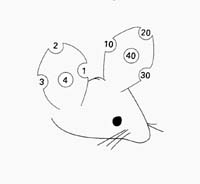# Ear Notch System

There are different ear notch systems. The CMGM uses the one described in Manipulating the Mouse Embryo - A Laboratory Manual. This system allows mice to be numbered from 1 to 99.

Reference
Hogan B, Beddington R, Costantini F and Lacy E, ed. 1994. Manipulating the Mouse Embryo - A Laboratory Manual, 2nd ed. Cold Spring Harbor Laboratory Press.

The CMGM Database uses the following 3 numbers to designate mice:

Breeding unit

Litter number

Mouse (ear notch) number

Example:  190578-3,2

Breeding unit is 190578

Litter number is 3

Mouse number is 2 and will have a “2” ear notch5 = 1 + 4
6 = 2 + 4
7 = 3 + 4
8 = 1 + 2
9 = 2 + 3

50 = 10 + 40
60 = 20 + 40
70 = 30 + 40
80 = 10 + 20
90 = 20 + 30